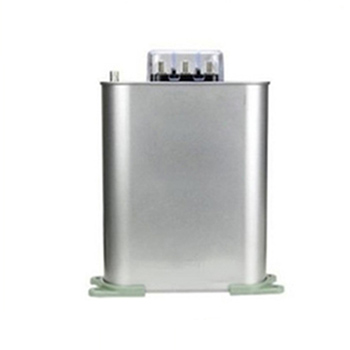Close
(0) Shopping cart
You have no items in your shopping cart.
Shopping Categories
Search

# Shunt Capacitor vs. Serial Capacitor

We often hear shunt capacitors and series capacitors in circuit devices. So what is the difference between them? Today, we will introduce the differences between series capacitors and shunt capacitors.

## What is a series capacitor?

A series capacitor is a reactive power compensation device. Usually series capacitors are used in EHV lines of 330kV and above. Its main function is to improve the system voltage from the perspective of compensating reactance, so as to reduce the power loss and improve the stability of the system. Capacitors must be able to withstand external forces due to short circuits, wind, snow and earthquakes. These mechanical forces are calculated through precise component analysis.

## What is a shunt capacitor?

The main function of shunt compensation capacitor for reactive power compensation is to reduce apparent current, improve power factor, reduce loss, and improve active power of power equipment.

From another point of view, the reactive power compensation of shunt capacitors can be understood as the use of capacitors to provide the required reactive current for electrical equipment, thereby reducing the burden on power lines, transformers and generators.## differences between shunt capacitors and series capacitor

The perspective of voltage regulation

The shunt capacitor improves the power factor of the load side to reduce the flow of reactive power to increase the voltage at the receiving end. According to the change of the load, the capacitors need to be switched on or off frequently in groups. The capacity of a capacitor is proportional to the square of the voltage. When the grid voltage drops, the voltage regulation effect drops significantly.

The voltage drop of the series capacitor is directly compensated for the line voltage drop, and the voltage regulation function is automatically and continuously adjusted with the change of the load. Its voltage regulation effect is much more significant than that of shunt capacitors. Therefore, only from the perspective of voltage regulation, only when the power factor is large, the ratio of line reactance to load impedance is also large. And the unit capacity price of series capacitors is larger than that of shunt capacitors. Therefore, the use of shunt capacitors is more beneficial.

The perspective of reducing network loss

After installing shunt capacitors, since the reactive power transmission capacity of transmission lines and transformers is reduced, shunt capacitor is much better than series capacitor, so it has a great effect on reducing network loss.

At the same time, the shunt capacitor can also transmit more active power with the reduced reactive power under the condition that the maximum transmission current of the transmission line remains unchanged. However, the series capacitance compensation basically does not change the reactive power transmission capacity on the transmission line, but only increases the terminal voltage level.

Therefore, the series compensation capacitor not only cannot reduce the network loss, but instead increases the load consumption and increases the line loss due to the increase of the load voltage. Series compensation will produce abnormal phenomena such as ferromagnetic resonance and self-excitation, which will cause harm to electrical equipment. In the ultra-high voltage transmission line, the series capacitor and the generator set may produce a sub-synchronous oscillation lower than the power frequency, which will cause the shaft torsional vibration and cause the generator shaft system damage.

Therefore, in the current grid below 220kV, the practical application of series capacitor compensation is less than that of shunt capacitor.

The equivalent capacitance is different

The equivalent capacitance of a shunt capacitor is equal to the sum of the capacitances in the capacitor bank, while the reciprocal of the equivalent capacitance of a series capacitor is equal to the sum of the reciprocals of the capacitances in the capacitor bank.

The equivalent capacitance of a series capacitor is smaller than the capacitance of any one capacitor in the capacitor bank. The equivalent capacitance of a shunt capacitor is larger than the capacitance of any capacitor in the capacitor bank.

The difference in capacity

When the capacitors are connected in series, the capacity decreases (for the calculation of the total capacity after connecting in series, refer to the parallel method of resistors), and the withstand voltage increases. When the capacitors are connected in parallel, the capacity increases (adding each capacity), and the withstand voltage is calculated as the smallest.

Series capacitors: The more the number of series, the smaller the capacitance, but the higher the withstand voltage. Its capacity relationship: 1/C=1/C1+1/C2+1/C3.

Shunt capacitors: The more the number of parallel connections, the greater the capacitance, but the withstand voltage remains unchanged, the capacity relationship: C=C1+C2+C3.